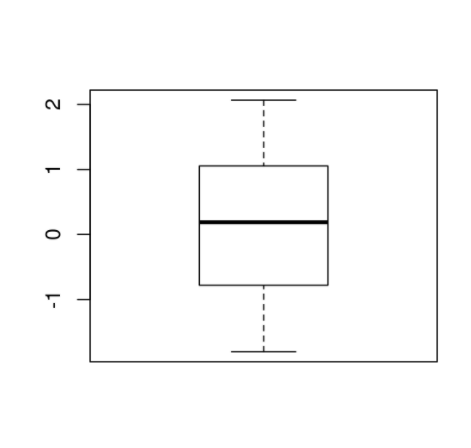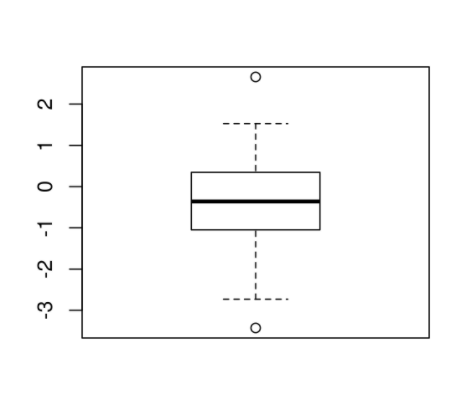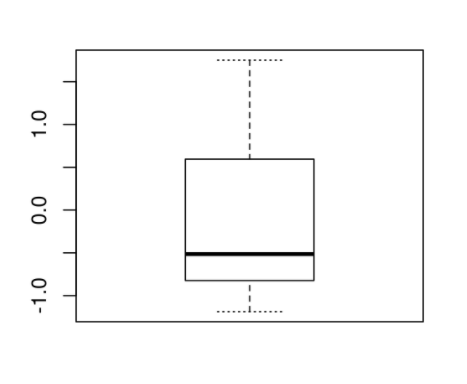# How to change the border line type in base R boxplot?

The default boxplot in R has straight border line type that display end point(s) excluding outliers. To change these border lines from a boxplot, we can use staplelty argument.

For Example, if we have a vector called X then we can create the boxplot of X with different border line type by using the command boxplot(X,staplelty=15). The argument can take different values. Check out the below Examples to understand how it works.

## Example

Consider the below vector −

x<-rnorm(15)

To create a boxplot of x, add the following code to the above snippet −

x<-rnorm(15)
boxplot(x)

## Output

If you execute the above given snippet, it generates the following Output −To create a boxplot of x with different border lines, add the following code to the above snippet −

x<-rnorm(15)
boxplot(x,staplelty=2)

## Output

If you execute all the above given snippets as a single program, it generates the following Output −Add the following code to the above snippet −

x<-rnorm(15)
boxplot(x,staplelty=15)

## Output

If you execute all the above given snippets as a single program, it generates the following Output −